# Gnuplotting

Create scientific plots using gnuplot

August 22nd, 2013 | 30 Comments

On the PGF plots page I found a nice example of visualizing data with cubes. Here we will recreate the same with gnuplot as you can see in Fig. 1.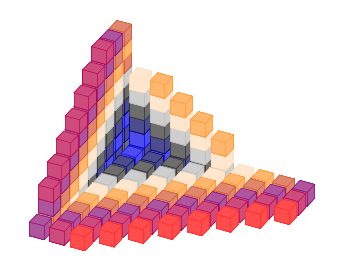Fig. 1 Cubes with different colors. (code to produce this figure, cube function, data)

We need basically two things in order to achieve it. First we have to plot a single cube with gnuplot. This is not as straight forward as you may think. We have to define a data file for it and plot it with the `pm3d` style which will result in Fig. 2.

```# single cube
0 0 0
0 0 1
0 1 1
0 1 0
0 0 0

1 0 0
1 0 1
1 1 1
1 1 0
1 0 0

0 0 0
1 0 0
1 1 0
0 1 0
0 0 0

0 0 1
1 0 1
1 1 1
0 1 1
0 0 1
```
```set cbrange [0.9:1]
set palette defined (1 '#ce4c7d')
set style line 1 lc rgb '#b90046' lt 1 lw 0.5
set pm3d depthorder hidden3d
set pm3d implicit
unset hidden3d
splot 'cube.txt' u 1:2:3:(1) w l ls 1
```

The use of the fourth `(1)` column for the `splot` command ensures that the cube gets the same color on every side. Only the edges are highlighted by a slighty different color given by the line style.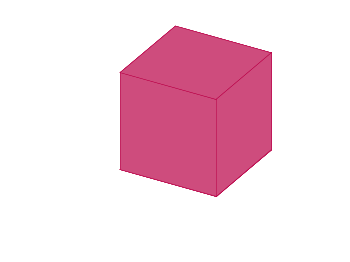Fig. 2 A single cube. (code to produce this figure, data)

In a second step we reuse the code from the Object placement using a data file entry in order to plot cubes at different positions with different colors. To get the different colors and positions we replace the cube.txt file with a cube function that returns the values for the desired position and color.

```add_cube(x,y,z,c) = sprintf('cube(%f,%f,%f,%i) w l ls %i,',x,y,z,c,c)
CMD = ''
stats 'cube_positions.txt' u 1:(CMD = CMD.add_cube(\$1,\$2,\$3,\$4)) nooutput
CMD = 'splot '.CMD.'1/0 w l ls 2'
eval(CMD)
```

December 18th, 2012 | 48 Comments

More than a year ago, we draw a map of the world with gnuplot. But it has been pointed out the low resolution of the map data. For example, Denmark is totally missing in the original data file. The good thing is, there is other data available in the web. In this entry we will consider how to use the physical coastline data from http://www.naturalearthdata.com/downloads/ together with gnuplot.Fig. 1 Plot of the world with the new data file and a resolution of 1:110m (code to produce this figure, data)

At the download page, three different resolutions of the data are available with a scale of 1:10m, 1:50m, or 1:110m. The data itself is stored as shape files on naturalearthdata.com. Hence I wrote a script that converts the data first to a csv file using the ogr2ogr program. Afterwards the data is shaped with sed into the form of the original world.dat file.
Here are the resulting files:

Fig.1 shows the result for a resolution of 1:110m. Note that we have to use linetype instead of linestyle in gnuplot 4.6 in order to set the colors of the grid and coast line.

In order to compare the different resolutions of the coast line files, we plot only the part of the map where Denmark is located (which is completely missing in the original data).
Here is the example code for a scale of 1:110m.

```set xrange [7.5:13]
set yrange [54.5:58]
plot 'world_110m.txt' with filledcurves ls 1, \
'' with l ls 2
```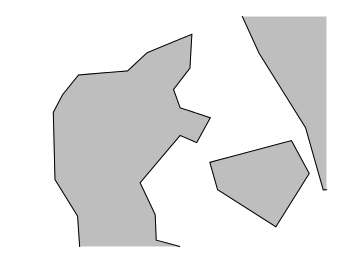Fig. 2 A plot of Denmark at a resolution of 1:110m. (code to produce this figure, data)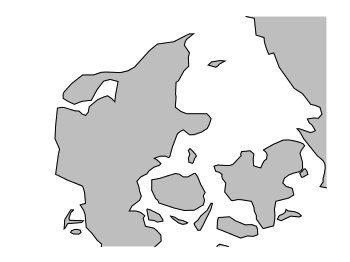Fig. 3 A plot of Denmark at a resolution of 1:50m. (code to produce this figure, data)Fig. 4 A plot of Denmark at a resolution of 1:10m. (code to produce this figure, data)

July 29th, 2011 | 5 Comments

In one post we have used the parametric plot option to plot the world. Here we want to add some temperature data as a heat map to the world plots. The data show the temperature anomalies of the year 2005 in comparison to the baseline 1951-1980 and is part of the GISTEMP data set.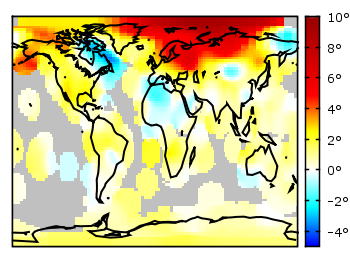Fig. 1 A 2D heat map of the temperature anomalies in 2005 to the baseline 1951-1980 (code to produce this figure, temperature data, world data)

The first problem you face, if you want to create a heat map, is that the data has to be in a specific format shown in the Gnuplot example page for heat maps. Therefore we first arrange the data and end up with this temperature anomalies file. Unknown data points are given by 9999.0.

In order to plot this data to the 2D world map we have to add a reasonable `cbrange` and a color `palette` and the plot command for the map:

```set cbrange [-5:10]
set palette defined (0 "blue",17 "#00ffff",33 "white",50 "yellow",\
66 "red",100 "#990000",101 "grey")
plot 'temperature.dat' u 2:1:3 w image, \
'world.dat' with lines linestyle 1
```

The trick with the wide range from 0 to 101 for the color bar is chosen in order to use `grey` for the undefined values (9999.0) without seeing the grey color in the color bar. The result is shown in Fig. 1.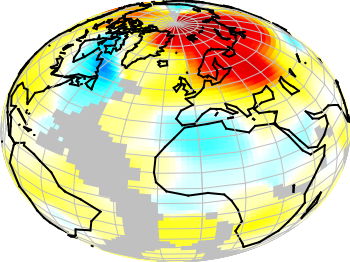Fig. 2 A 3D heat map of the temperature anomalies in 2005 to the baseline 1951-1980 (code to produce this figure, temperature data, world data)

The same data can easily be applied to the 3D plot of the world. We have to add `front` to the `hidden3d` command in order to make the black world lines visible. In addition the radius must be given explicitly as third column to the plot command for the temperature data.

```set hidden3d front
splot 'temperature.dat' u 2:1:(1):3 w pm3d, \
r*cos(v)*cos(u),r*cos(v)*sin(u),r*sin(v) w l ls 2, \
'world.dat' u 1:2:(1) w l ls 1
```

The result is shown in Fig. 2.

June 7th, 2011 | 6 Comments

A Klein bottle is a non-orientable surface, which has no defined left and right, as stated on Wikipedia. There we can also find a Gnuplot plot of the bottle, which we want to fine-tune a little bit in order to reach the result in Fig. 1.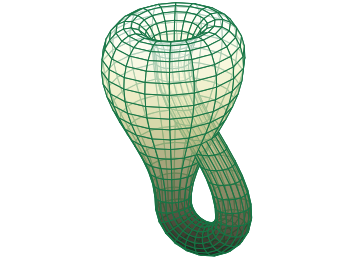Fig. 1 Klein bottle (code to produce this figure)

In order to reach Fig. 1 we start with the definition of the parametric functions given in Wikipedia and do a simple `pm3d` plot with them.

```set parametric
x(u,v)= v<2*pi ? (2.5-1.5*cos(v))*cos(u) : \
v<3*pi ? -2+(2+cos(u))*cos(v)    : \
-2+2*cos(v)-cos(u)
y(u,v)= v<2*pi ? (2.5-1.5*cos(v))*sin(u) : \
sin(u)
z(u,v)= v<pi   ? -2.5*sin(v)             : \
v<2*pi ? 3*v-3*pi                : \
v<3*pi ? (2+cos(u))*sin(v)+3*pi  : \
-3*v+12*pi
splot x(u,v),y(u,v),-z(u,v) w pm3d
```

The result is shown in Fig. 2.Fig. 2 Klein bottle plotted only with pm3d (code to produce this figure)

Now we add some lines to the surface and hide parts, which are not visible in 3d.

```set pm3d depthorder hidden3d 1
set hidden3d
```

Here the `depthorder` option takes care of the right positioning of the bottleneck going back into the bottle, which is not correct in Fig. 2. The `hidden3d 1` option draws lines in the right order for a correctly looking 3d plot using line style 1. The additional `set hidden3d` command takes care of showing only those lines that are visible in 3d. These options will result in Fig. 3.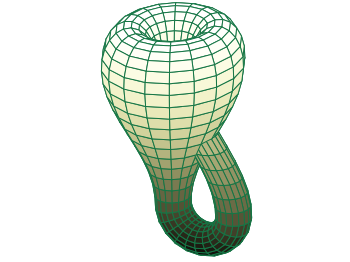Fig. 3 Klein bottle plotted only with pm3d and hidden3d (code to produce this figure)

In order to reach at Fig. 1 we just have to set the surface to be transparent, which can be done by the `set style fill` command.

```set style fill transparent solid 0.65
```